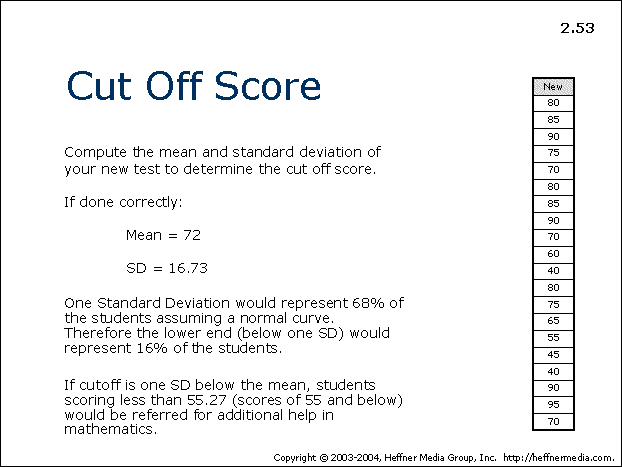advertisement

# 53• Compute the mean and standard deviation of your new test to determine the cut off score.
• If done correctly:
• Mean=72
• SD=16.73
• One Standard Deviation would represent 68% of the students assuming a normal curve. Therefore the lower end (below one SD) would represent 16% of the students.
• If cutoff is one SD below the mean, students scoring less than 55.27 (scores of 55 and below) would be referred for additional help in mathematics.